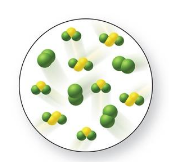# Problem: Consider the reaction shown below with its equilibrium constant:            S 2Cl 2(g) + Cl 2(g) ---&gt;  2SCl 2(g)  Keq = 4Examine the figure, and determine if the system is at equilibrium. If it is not, in which direction will it proceed to reach equilibrium?    A)    The reaction is at equilibrium.    B)    The reaction is not at equilibrium, it will shift to the left.    C)    The reaction is not at equilibrium, it will shift to the right.    D)    It is not possible to tell if the reaction is at equilibrium.    E)    To reach equilibrium, the value of Keq must change

###### Problem Details

Consider the reaction shown below with its equilibrium constant:

S 2Cl 2(g) + Cl 2(g) --->  2SCl 2(g)  Keq = 4

Examine the figure, and determine if the system is at equilibrium. If it is not, in which direction will it proceed to reach equilibrium?

A)    The reaction is at equilibrium.

B)    The reaction is not at equilibrium, it will shift to the left.

C)    The reaction is not at equilibrium, it will shift to the right.

D)    It is not possible to tell if the reaction is at equilibrium.

E)    To reach equilibrium, the value of Keq must change